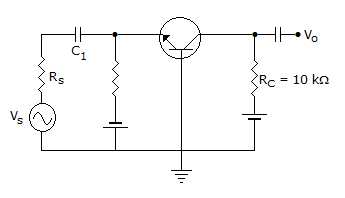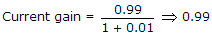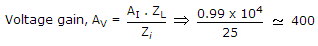# Electronics and Communication Engineering - Analog Electronics - Discussion

40.

The 'h' parameters of the circuit shown in the figure are hib = 25 Ω, hfb = 0.999 and hob = 10-6Ω The Voltage gain is[A]. 0.99 [B]. 1.98 [C]. 2 [D]. 4

Explanation:Zi = 25 + 10-6 x 0.99 x 10425.

 Abhi said: (Aug 5, 2016) Can't understand How? Explain clearly.

 Arun said: (Oct 12, 2016) Please explain the solution in right way.

 Ajit Dubey said: (Nov 11, 2016) For approx calculations of CE amplifier input impedance Ri = hib = 25 and c is shorted for ac analysis, for current gain Ai = Hfb = 0.99. So put all this in Av = Ai * RL/his. Hope this helps.

 Liza said: (Jun 5, 2017) How can we get the value of zL?

 Subbu said: (Jul 25, 2017) zL is load resistance or effective resistance seen a/c the load hence zL=Rc=10k=10^4.